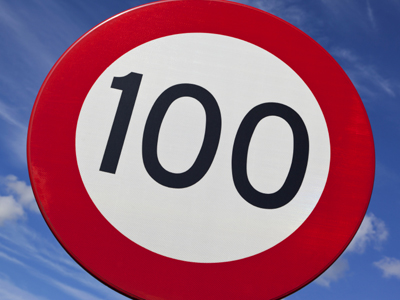The value of the digit 1 in 420,108 is 100.

# Place Value 3

This Math quiz is called 'Place Value 3' and it has been written by teachers to help you if you are studying the subject at elementary school. Playing educational quizzes is an enjoyable way to learn if you are in the 3rd, 4th or 5th grade - aged 8 to 11.

It costs only \$12.50 per month to play this quiz and over 3,500 others that help you with your school work. You can subscribe on the page at Join Us

The values of digits change depending on their place in a number. A two, for example, can be worth 2 units, 2 tens, 2 hundreds or 2 thousands, the equivalent of 2, 20, 200 and 2,000. So, which number would be the equivalent of 4 hundreds, 3 tens and 5 units? The answer is 435.

1.
Which has the smallest value?
40,000
41 thousands
410 hundreds
4,100 tens
All the numbers are equivalent to 41,000 except 40,000 which is the smallest
2.
Which is the number that is equivalent to sixty-two thousand seven hundred and eighty-two?
62,782
620,872
628,072
627.82
62,782 has 6 ten thousands
2 one thousands
7 hundreds
8 tens
and 2 units
3.
Which is 48,921 in words?
Four hundred thousand eighty nine hundred and twenty one
Forty eight thousand nine hundred and twenty one
Forty eight thousand and twenty one
Four hundred and eight thousand nine hundred and twenty one
48,921 has 4 ten thousands
8 one thousands
9 hundreds
2 tens
and 1 unit
4.
What is the value of the digit 1 in 420,108?
100
1,000
10,000
100,000
The 1 is in the hundreds column so is worth 1 x 100 = 100
5.
What is the value of the digit 9 in 28,945?
90
900
9,000
90,000
The 9 is in the hundreds column so is worth 9 x 100 = 900
6.
What needs to be subtracted from 19,723 to make 12,723?
70
700
7,000
70,000
The digit 9 is equivalent to 9,000 so to change it to 2,000 you must subtract 7,000
7.
Which is the number that is equivalent to three hundred and seventy-four thousand and fifty-two?
37,452
3,074,052
307,452
374,052
374,052 has 3 hundred thousands
7 ten thousands
4 one thousands
0 hundreds
5 tens
and 2 units
8.
What does the digit 3 in 36,218 represent?
30
300
3,000
30,000
The 3 is in the ten thousands column so is worth 3 x 10,000 = 30,000
9.
What does the digit 4 in 401,253 represent?
400
4,000
40,000
400,000
The 4 is in the hundred thousands column so is worth 4 x 100,000 = 400,000
10.
What needs to be added to 45,612 to make 45,912?
30
300
3,000
30,000
The digit 6 is worth 600 so to change it to 900 you must add 300
Author:  Amanda Swift